# Intuition For Polynomials

Polynomials are those long equations you were forced to learn about in school: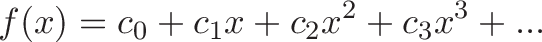$\displaystyle{f(x) = c_0 + c_1x + c_2x^2 + c_3x^3 + ... }$

We have a bunch of coefficients ($c_0, c_1...$ ) multiplied by various powers of $x$.

Why are we forced to learn about them? Here's the insights I wish I had.How many lines are here?

|||||||||||||||||||||||||||||||||||||||||||||||||||||||||||||||||||||||||||||||||||||||||||||||||||||||||||||||||||||||||||

Why it's one hundred twenty three, of course. Oh, you'd probably have preferred the number in its polynomial form: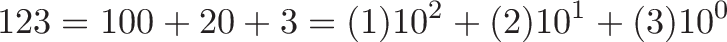$\displaystyle{123 = 100 + 20 + 3 = (1)10^2 + (2)10^1 + (3)10^0}$

The digits "123" are simply the coefficients for various powers of 10, written largest to smallest. The "simple" idea of counting by adding powers of 10 is pretty game-changing, right?

We can even switch bases. The general interpretation of "123", for any base, is: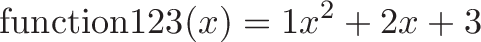$\displaystyle{\text{function123}(x) = 1x^2 + 2x + 3}$

We're already familiar with decimals, so turn the coefficients "123" into a quantity we can understand. For hexadecimal numbers, we plug in $x = 16$ and work out $(1)16^2 +(2)16 + (3)16^0 = 291$.

Strictly speaking, 123.4 involves negative powers, since $0.1 = 10^{-1}$. Still, the original notion of polynomials gave us a good start, better than counting with lines in the sand.

## Polynomials consolidate simple arithmetic

Imagine you're taking notes for someone who's too lazy to use a calculator. They might say:

"Take the number 15. Actually, add 3. Multiply by 7. Now multiply by the original number again."

As arithmetic, you might write: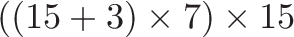$\displaystyle{((15 + 3) \times 7) \times 15 }$

This is an exact expression, with specific numbers. But maybe your buddy is indecisive.

Making this more general -- with any starting number -- we'd write: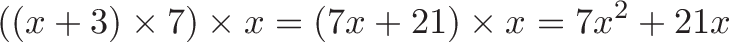$\displaystyle{((x + 3) \times 7) \times x = (7x + 21)\times x = 7x^2 + 21x }$

Notice that all the intermediate steps have been reduced to 2 terms. A polynomial!

Instead of thinking "When will I see this equation", think "This equation is the simplified version of a bunch of arithmetic". The steps don't look similar (where's the "add 3" portion?) but the polynomial boils everything to the essentials.

So, another big insight: we don't always need to solve a polynomial, we can use it to simplify many stages of arithmetic.

But... now that it's in its equation form, we could solve it. If we wanted to. Because it seems like a mathy thing to do.

Let's say we want to know when this process hits 100. What input number would that be?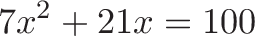$\displaystyle{7x^2 + 21x = 100}$

Solving this gives $x = -5.56$ and $x=2.56$. (Yes, in the real world we can use tools to solve equations; grinding through the quadratic formula is an intuition for another day.)

## Polynomials are simpler to study than arbitrary functions

The big aha is that polynomials are the boiled down version of arithmetic, assuming you can only:

• Add/subtract/multiply/divide by a constant, or:
• Multiply by your original number, x

Strictly speaking, polynomials don't allow for square roots ($\sqrt{x}$) or variable exponents ($2^x$), just the simple operations above.

Why limit ourselves this way? Well, we can see how far we can get.

Linear algebra is limited to linear functions, but even then we can model complex behavior if we chain enough of them together.

Similarly, polynomials are simple, but with enough terms we can model pretty complex behavior. The simple structure gives us several nice properties:

• Adding/multiplying polynomials gives us a polynomial
• Divide a polynomial by its roots, $(x - r)$, and get a polynomial (like dividing a compound number by one of its factors)
• Feed polynomials into each other $f(g(x))$
• The derivative / integral of a polynomial is a polynomial, making them easy to optimize

Polynomials are like the integers -- simpler than real numbers, and still useful.

## Polynomials track interactions

What does $x$ mean? Well, it's a number, a quantity, something we want to represent.

What does $x \cdot x = x^2$ mean? It's an interaction: a quantity interacting with itself. In the case of length interacting with length, the result is area: length * length = square units. Or, we could have speed interacting with time to make distance: 30 mph for 30 hours is 900 miles.

A big aha: math doesn't care about units. A polynomial does the bookkeeping to show that an interaction like $x \cdot x$ happened, along with plain old $x$ and $x^{17}$.

In physics, you can't sensibly write $3cm + 5cm^2$ because the powers of the units don't match. You can't write $3 cm + 5kg$ because the types of units don't match.

But math doesn't care. Numbers are numbers, and "1 + 2*2 = 5" works, even though "2 times 2" represents an interaction.

A polynomial is a general-purpose accounting system where we can throw anything in the pot of soup. Will it taste good? Not the polynomial's problem. You'll get a formula and decide what, if anything, to do with it.

## Polynomials are the DNA inside a function

Given an input $x$, polynomials show what happens when we perform an ungodly amount of arithmetic to that input.

It seems, if we twiddle enough levers, we can get that polynomial to behave like almost any pattern we choose.

You got it: that's the Taylor series.

With enough terms, our humble polynomial -- composed of basic arithmetic -- can model undulating sine waves and other things that give students nightmares.

Each term in the polynomial gets us a better approximation of the original function: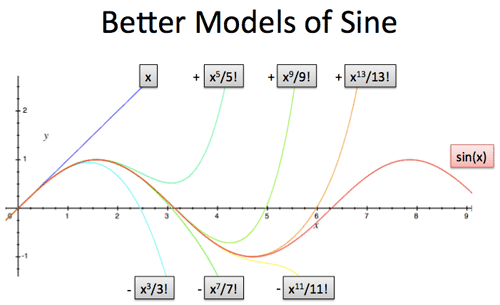We can analyze the coefficients in the polynomial and extract the "DNA" inside a function:$\displaystyle{ \sin x = x - \frac{x^3}{3!} + \frac{x^5}{5!} - \dots \xrightarrow{DNA} [0, 1, 0 -1, \dots] }$$\displaystyle{ \cos x = 1 - \frac{x^2}{2!} + \frac{x^4}{4!} - \dots \xrightarrow{DNA} [1, 0, -1, 0, \dots] }$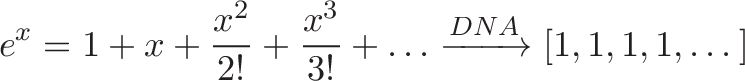$\displaystyle{ e^x = 1 + x + \frac{x^2}{2!} + \frac{x^3}{3!} + \dots \xrightarrow{DNA} [1, 1, 1, 1, \dots] }$

If the pattern in the coefficients are similar, the functions are likely related.

Back to the decimal analogy: 12 and 120 are similar, but that's only obvious when I write them as "decimal polynomials". If I spilled 12 and 120 skittles on the floor, you wouldn't notice any obvious connection between the two piles. Well, one might look slightly more appetizing.

## Polynomials turn addition to multiplication

One of the big insights in math -- called the Fundamental Theorem of Algebra -- is that a polynomial can be rewritten as a sequence of multiplications.

That is, adding powers of $x$ can also be seen as multiplying $x$ with various offsets: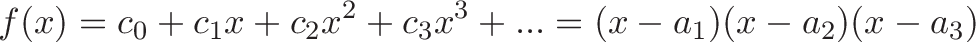$\displaystyle{f(x) = c_0 + c_1x + c_2x^2 + c_3x^3 + ... = (x - a_1)(x - a_2)(x - a_3)}$

There's as many roots ($a_1, a_2, a_3$) as the highest power of $x$ (roots can be used multiple times).

Why is this important? Well, besides being incredibly surprising (added powers can be converted to multiplications), it makes solving equations much easier (see: why do we factor equations?).

In short, it's not instantly obvious how to satisfy this: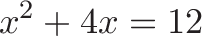$\displaystyle{x^2 + 4x = 12}$

Want to guess answers? Plug in $x$ and it shows up in places terms which need to be balanced. But what about this: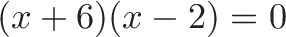$\displaystyle{(x + 6)(x - 2) = 0}$

It's the same scenario, factored into multiplications. If any term goes to zero, the entire product becomes zero and we're done. Just pick $(x + 6)$ or $(x - 2)$, make it zero ($x = -6$ or $x = 2$), and we've solved the puzzle.

Remember how "123" was secretly a polynomial? For fun, using the accursed quadratic formula, we can factor it: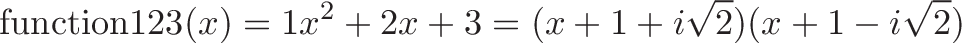$\displaystyle{\text{function123}(x) = 1x^2 + 2x + 3 = (x + 1 + i\sqrt{2})(x + 1 - i\sqrt{2})}$

What does this mean? We can get the digits "123" to equal zero if we use a complex base! Not that you'd want to... but it's possible.

## Polynomials are simple to optimize

A polynomial is simple: just a bunch of powers of x. That means it's easy to use the Power Rule in Calculus to find the derivative, and find the min/max.

The reverse works as well: we can take a bunch of data points, fit a polynomial to them, and estimate the min/max of the sequence. The more terms, the more accurate (but beware overfitting).

A simple structure has its advantages.

## Onward

Polynomials usually evoke memories of the quadratic equation, or equations force-fit into word problems. Ugh, let's skip past that.

Polynomials are a simple, powerful model used from arithmetic to algebra to calculus. They're as applicable in the "real world" as a 2-digit number.

Happy math.